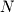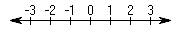Linear algebra is a branch in mathematics that deals with objects and operations in what is called-dimensional space. Most students are already familiar with 1, 2 and 3-dimensional spaces from elementary algebra. 1-dimensional space can be visualized as a number line as shown in Figure 1-1. The only objects are points which can be represented by a single numerical values.Figure 1-1.
Number Line
2-dimensional space is a flat plane and consists of primitive objects such as points and lines. Individual objects are combined to form more complicated ones such as the parallelogram in Figure 1-2. 3-dimensional space consists of points, lines, and planes. Once again, objects such as a parallelpiped (pronounced parallel-pipe-ed) can be formed from more primitive objects. Operations on objects range from finding the distance between two points to rotations and translations of the object.
 Object could not be loaded.Figure 1-2. Parallelogram in 2D Space Object could not be loaded.Figure 1-3. Parallelpiped in 3D Space